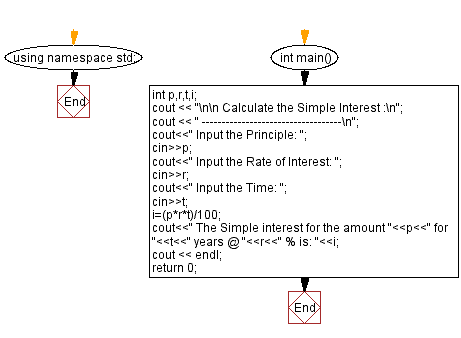﻿ C++ Exercises: Calculate Simple Interest - w3resource

# C++ Exercises: Calculate Simple Interest

## C++ Basic: Exercise-54 with Solution

Write a C++ program to enter P, T, R and calculate Simple Interest.

Sample Solution:

C++ Code :

``````#include<iostream>
using namespace std;

int main()
{
int p,r,t,i;
cout << "\n\n Calculate the Simple Interest :\n";
cout << " -----------------------------------\n";
cout<<" Input the Principle: ";
cin>>p;
cout<<" Input the Rate of Interest: ";
cin>>r;
cout<<" Input the Time: ";
cin>>t;
i=(p*r*t)/100;
cout<<" The Simple interest for the amount "<<p<<" for "<<t<<" years @ "<<r<<" % is: "<<i;
cout << endl;
return 0;
}
``````

Sample Output:

``` Calculate the Simple Interest :
-----------------------------------
Input the Principle: 20000
Input the Rate of Interest: 10
Input the Time: 1.5
The Simple interest for the amount 20000 for 1 years @ 10 % is: 2000
```

Flowchart:C++ Code Editor:

What is the difficulty level of this exercise?

﻿

## C++ Programming: Tips of the Day

Why is there no std::stou?

The most pat answer would be that the C library has no corresponding "strtou", and the C++11 string functions are all just thinly veiled wrappers around the C library functions: The std::sto* functions mirror strto*, and the std::to_string functions use sprintf.

Ref: https://bit.ly/3wtz2qA

We are closing our Disqus commenting system for some maintenanace issues. You may write to us at reach[at]yahoo[dot]com or visit us at Facebook